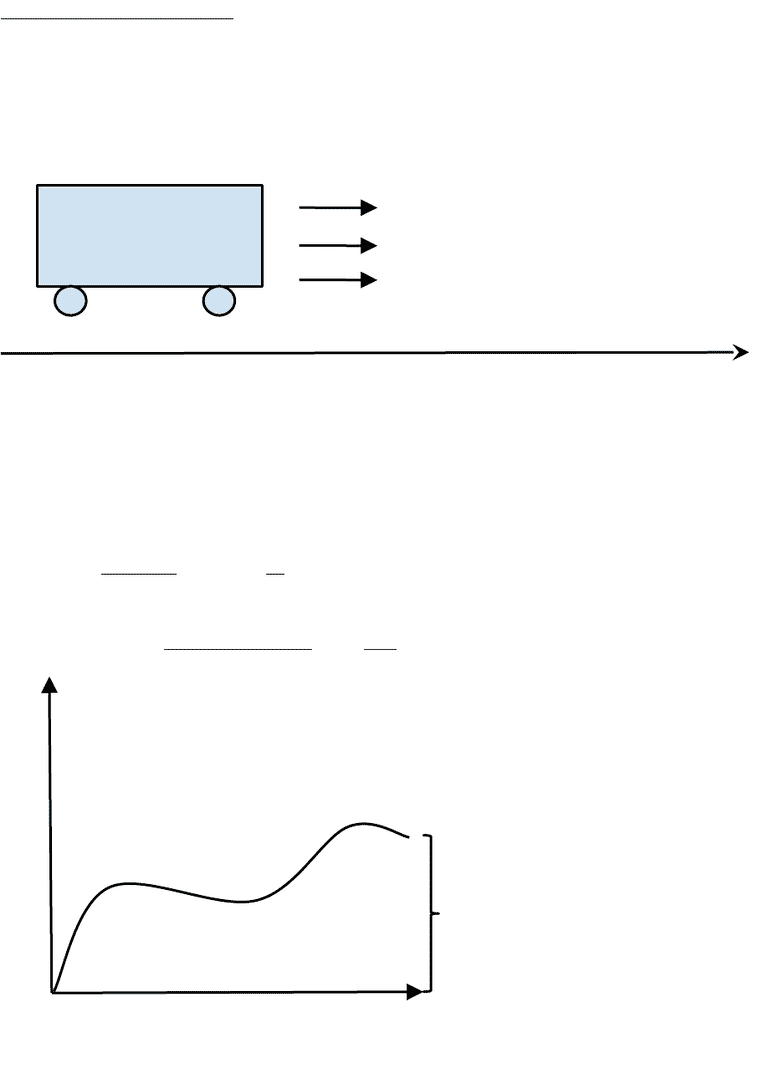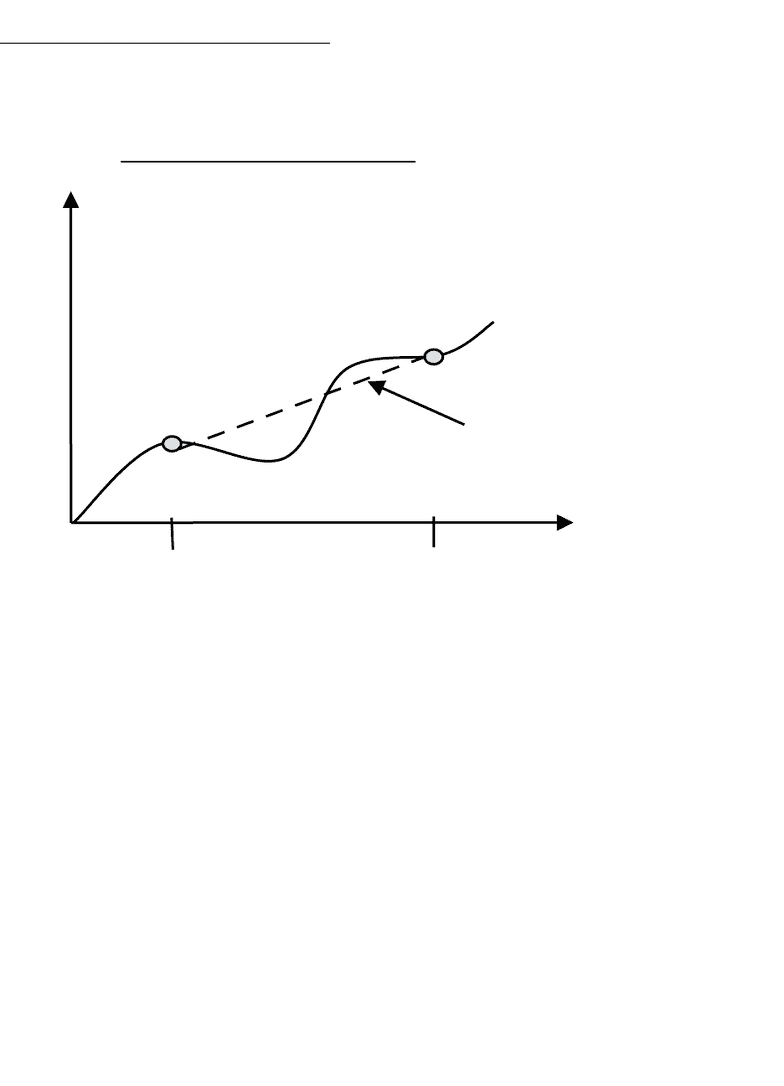Get 2 days of unlimited access
Study Guides (350,000)
US (190,000)
BU (5,000)
CAS MA (100)
Final

# CAS MA 123 Study Guide - Final Guide: Quadratic Equation, Orcus, Sox2Premium

Department
Mathematics & Statistics
Course Code
CAS MA 123
Professor
John Bergdall
Study Guide
Final

This preview shows pages 1-3. to view the full 65 pages of the document.MA 123 – Calculus I
Final Exam Study Guide
University of Boston – Fall 2015

Only pages 1-3 are available for preview. Some parts have been intentionally blurred.

Subscribers OnlyMA 123 - Lecture 1 - Limits
Limits:
developed in the 19th century by Cauchy
measure both velocity and area which are defined using limits and approximations
fundamental theorem of calculus correlates to the rate of change = area
computing velocity is a basic problem
Question 1:
From start to end, how fast was the car going?
Velocity =
Distance
Time
or V =
D
T
Average Velocity=
Distance Traveled
Time Elapsed
=
rise
run
= slope of the line
Car is moving
Distance
Distance
Traveled
Positio
n
Time

Only pages 1-3 are available for preview. Some parts have been intentionally blurred.

Subscribers OnlyMA123 - Lecture 2 - Limits Continued
Average Velocity:
Take an equation of motion P(t)
The average velocity from time #1 to time #2 equals
position at time 2 - position at time 1
time 2 - time 1
Question:
How do you compute instantaneous velocity?
The idea is to fix one time of interest, T0, let the second time vary
The instantaneous velocity at T0 is approximately the average velocity from time T0 to
another time.
P(t
)
t
T1 T2
(T1,P(T1)) Slope = Average Velocity
###### You're Reading a Preview

Unlock to view full version

Subscribers Only